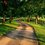# [Differential Geometry] What does an Asymptotic Geodesic Line of Curvature look like?

How could we show that a curve $C \subset S$ on a smooth, orientable surface is both an asymptotic curve and a geodesic if and only if C is a segment of a straight line?

$\Rightarrow$ NTS: If $C$ is both asymptotic and a geodesic, then it is a straight line.

By definition, asymptotic curve of a regular surface is such that, the tangent line of C at each point of the curve is an asymptotic direction, and along asymptotic directions the normal curvature is zero. Hence $k_n = 0$. The relationship of normal, geodesic, and usual curvature of $C$ gives: $(k_n)^2 + (k_g)^2=k^2.$ And since we have $k_n=0$, $\mid k_g \mid = k.$

Additionally, a curve is geodesic if and only if $k_g = 0$ at each point of the curve. So $k=0$. Since the usual curvature of curve C is zero at all points, if we let $\alpha(s)$ be a regular parametrisation of C, we have that $\vert \alpha ''(s) \vert \equiv 0$. Then by integration, $\alpha(s) = bs + c$, so the curve is a (segment of) a straight line.

$\Leftarrow$ NTS: If $C$ is a straight line, then it is both an asymptotic curve and a geodesic.

If $C$ is a straight line, the usual curvature of $C$ is zero, hence $k=0$. From the relation $(k_n)^2+(k_g)^2=k^2$, we see that $(k_n)^2 \geq 0$, and $(k_g)^2 \geq 0$, so $k_n$ and $k_g$ must both be zero. Therefore, C is both asymptotic and a geodesic line of curvature.

Therefore, we conclude that $C \subset S$ is asymptotic and geodesic curve if and only if (C) is a (segment of) a straight line.Note by Bright Glow
3 years, 2 months ago

This discussion board is a place to discuss our Daily Challenges and the math and science related to those challenges. Explanations are more than just a solution — they should explain the steps and thinking strategies that you used to obtain the solution. Comments should further the discussion of math and science.

When posting on Brilliant:

• Use the emojis to react to an explanation, whether you're congratulating a job well done , or just really confused .
• Ask specific questions about the challenge or the steps in somebody's explanation. Well-posed questions can add a lot to the discussion, but posting "I don't understand!" doesn't help anyone.
• Try to contribute something new to the discussion, whether it is an extension, generalization or other idea related to the challenge.

MarkdownAppears as
*italics* or _italics_ italics
**bold** or __bold__ bold
- bulleted- list
• bulleted
• list
1. numbered2. list
1. numbered
2. list
Note: you must add a full line of space before and after lists for them to show up correctly
paragraph 1paragraph 2

paragraph 1

paragraph 2

[example link](https://brilliant.org)example link
> This is a quote
This is a quote
    # I indented these lines
# 4 spaces, and now they show
# up as a code block.

print "hello world"
# I indented these lines
# 4 spaces, and now they show
# up as a code block.

print "hello world"
MathAppears as
Remember to wrap math in $$ ... $$ or $ ... $ to ensure proper formatting.
2 \times 3 $2 \times 3$
2^{34} $2^{34}$
a_{i-1} $a_{i-1}$
\frac{2}{3} $\frac{2}{3}$
\sqrt{2} $\sqrt{2}$
\sum_{i=1}^3 $\sum_{i=1}^3$
\sin \theta $\sin \theta$
\boxed{123} $\boxed{123}$